Electrostatics NAT Level - 2

# Electrostatics NAT Level - 2

Test Description

## 10 Questions MCQ Test Topic wise Tests for IIT JAM Physics | Electrostatics NAT Level - 2

Electrostatics NAT Level - 2 for IIT JAM 2023 is part of Topic wise Tests for IIT JAM Physics preparation. The Electrostatics NAT Level - 2 questions and answers have been prepared according to the IIT JAM exam syllabus.The Electrostatics NAT Level - 2 MCQs are made for IIT JAM 2023 Exam. Find important definitions, questions, notes, meanings, examples, exercises, MCQs and online tests for Electrostatics NAT Level - 2 below.
Solutions of Electrostatics NAT Level - 2 questions in English are available as part of our Topic wise Tests for IIT JAM Physics for IIT JAM & Electrostatics NAT Level - 2 solutions in Hindi for Topic wise Tests for IIT JAM Physics course. Download more important topics, notes, lectures and mock test series for IIT JAM Exam by signing up for free. Attempt Electrostatics NAT Level - 2 | 10 questions in 45 minutes | Mock test for IIT JAM preparation | Free important questions MCQ to study Topic wise Tests for IIT JAM Physics for IIT JAM Exam | Download free PDF with solutions
 1 Crore+ students have signed up on EduRev. Have you?
*Answer can only contain numeric values
Electrostatics NAT Level - 2 - Question 1

### Figure shows a solid hemisphere with a charge of 5nC  distributed uniformly throughout its volume. The hemisphere lies on a plane and point P is located on the plane, along a radial line from the centre of curvature at distance 15cm. The electric potential at point P (in volts) due to the hemisphere, is___.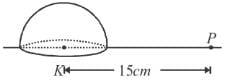Detailed Solution for Electrostatics NAT Level - 2 - Question 1

By argument of symmetry, it will be half of the potential produced by the full sphere

∵ – Charge on hemisphere = Q, so charge on sphere = 2Q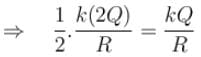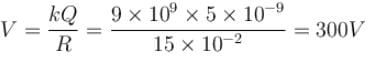*Answer can only contain numeric values
Electrostatics NAT Level - 2 - Question 2

### A simple pendulum with a bob of mass m = 1kg, charge q = 5μC and string length l = 1m is given a horizontal velocity u in a uniform electric field E = 2 × 106 V/m  at its bottom most point A, as shown in figure. It is given that the speed u is such that the particle leaves the circle at point C. Find the speed u.  (Take g = 10m/s2)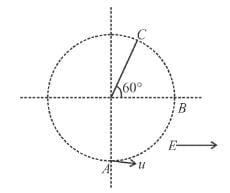Detailed Solution for Electrostatics NAT Level - 2 - Question 2

From work-energy theorem,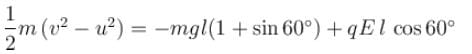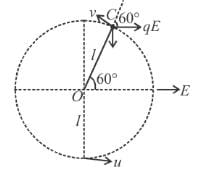Substituting the values, we get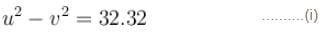Further, at C tension in the string is zero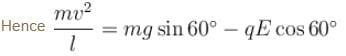or  v2 = 3.66 ......(ii)
From Eqs. (i) and (ii), we get

u = 6m/s

*Answer can only contain numeric values
Electrostatics NAT Level - 2 - Question 3

### A position charge +Q is fixed at a point A. Another positively charged. particle of mass m and charge +q is projected from a point B with velocity u as shown in the figure. The point B is large distance from A and at distance d from the line AC. The initial velocity is parallel to the line AC. The point C is at very large distance from A. Find the minimum distance (in meter) of +q from +Q during the motion. Take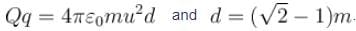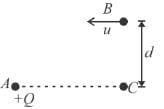Detailed Solution for Electrostatics NAT Level - 2 - Question 3

The path of the particle will be as shown in the figure. At the point of minimum distance (D) the velocity of the particle will be to its position vector w.r.t +Q.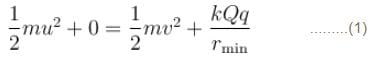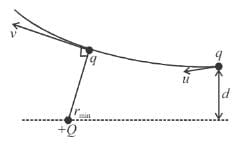∵ Torque on q about Q is zero hence angular momentum about Q will be conserved
⇒  mvrmin = mud      ........(2)
by (2) in (1)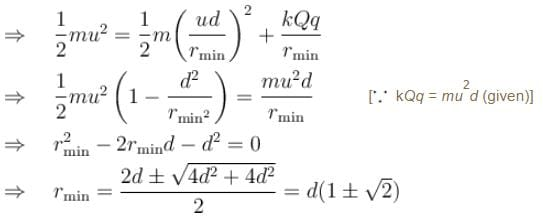∵   distance cannot be negative
∴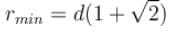*Answer can only contain numeric values
Electrostatics NAT Level - 2 - Question 4

The electric potential varies in space according to the relation V = 3x + 4y. A particle of mass 10kg starts from rest from point (2,3.2)m under the influence of this field. Find the velocity of the particle in units of 10–3m/s, when it crosses the x-axis. The charge on the particle is 1μC. Assume V(x,y) are in SI units.

Detailed Solution for Electrostatics NAT Level - 2 - Question 4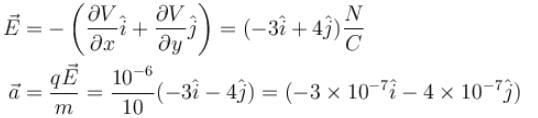When particle crosses x-axis, y = 0

Initial y-coordinate was 3.2 m and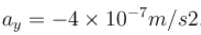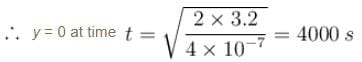At this instant x-coordinate will be,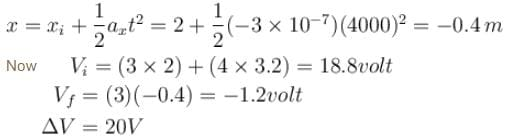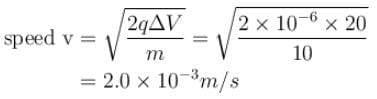*Answer can only contain numeric values
Electrostatics NAT Level - 2 - Question 5

Consider a cube of side a = 0.1m  placed such that its six faces are given by equations x = 0, x = + a, y = 0, y = +a, z = 0 and z = +a, placed in electric field given by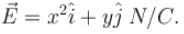Find the electric flux crossing out of the cube in the unit of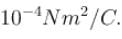Detailed Solution for Electrostatics NAT Level - 2 - Question 5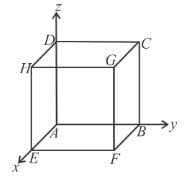Flux through ABCD.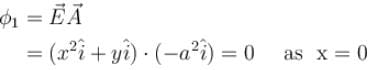Flux through BCGF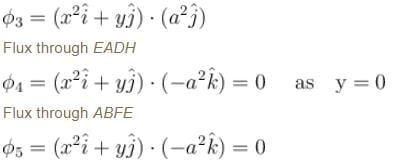Flux through CDHG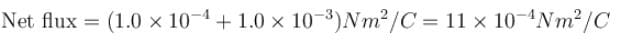*Answer can only contain numeric values
Electrostatics NAT Level - 2 - Question 6

A solid sphere of radius R has a cavity of radius R/2 The solid part has a uniform charge density ρ and cavity has no charge. The electric potential at point A is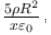then value of x is

Detailed Solution for Electrostatics NAT Level - 2 - Question 6

Assume a solid sphere without cavity.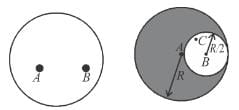Potential at A due to this solid sphere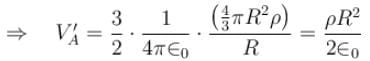Electric field at C due to this solid sphere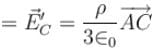Now consider the cavity filled with negative charge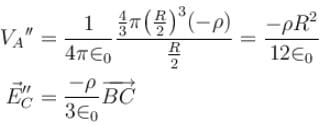Now net values for the solid sphere with the cavity can be given by superposition of the above two cases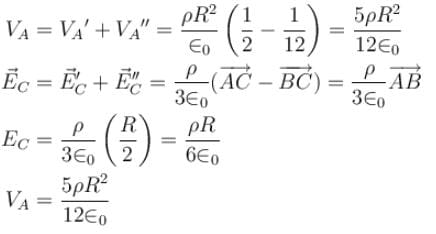*Answer can only contain numeric values
Electrostatics NAT Level - 2 - Question 7

A graph of the x component of the electric field as a function of x in a region of space is shown. The y and z components of the electric field are zero in this region. If the electric potential is 10V at the origin, then potential (in volts) at x = 2.0m is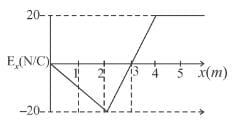Detailed Solution for Electrostatics NAT Level - 2 - Question 7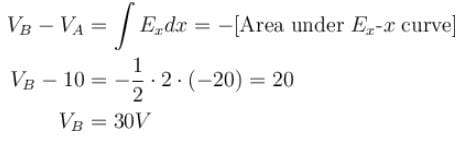*Answer can only contain numeric values
Electrostatics NAT Level - 2 - Question 8

A dipole of dipole moment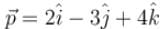is placed at point A(2, -3, 1). The electric potential due to this dipole at the point B(4, -1, 0) is equal to n × 109 volts. Find the value of n.

Detailed Solution for Electrostatics NAT Level - 2 - Question 8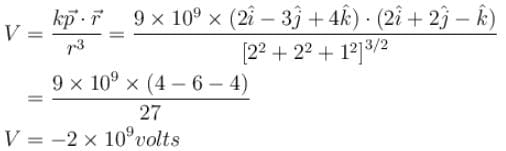*Answer can only contain numeric values
Electrostatics NAT Level - 2 - Question 9

A 4.00 kg block carrying a charge Q = 50.0μC is connected to a spring for which k = 100N/m. The block lies on a friction less horizontal track, and the system is immersed in a uniform electric field of magnitude E = 5.00 × 105 V/m, directed as shown in figure. If the block is released from rest when the spring is unscratched (at x = 0). By what maximum amount does the spring expand. (Answer in meters)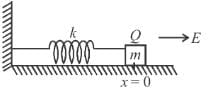Detailed Solution for Electrostatics NAT Level - 2 - Question 9

By comparing this problem with spring-block system problem suspended vertically.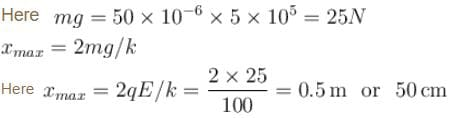*Answer can only contain numeric values
Electrostatics NAT Level - 2 - Question 10

Three identical, conducting plane parallel plates, each of area A are held with equal separation d between successive surfaces. Charges Q, 2Q, and 3Q are placed on them. Neglecting edge effects. The sum of charges on the six surfaces is given by nQ. Find the value of n.

Detailed Solution for Electrostatics NAT Level - 2 - Question 10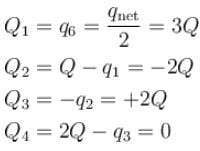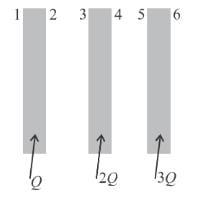## Topic wise Tests for IIT JAM Physics

217 tests
 Use Code STAYHOME200 and get INR 200 additional OFF Use Coupon Code
Information about Electrostatics NAT Level - 2 Page
In this test you can find the Exam questions for Electrostatics NAT Level - 2 solved & explained in the simplest way possible. Besides giving Questions and answers for Electrostatics NAT Level - 2, EduRev gives you an ample number of Online tests for practice

217 tests